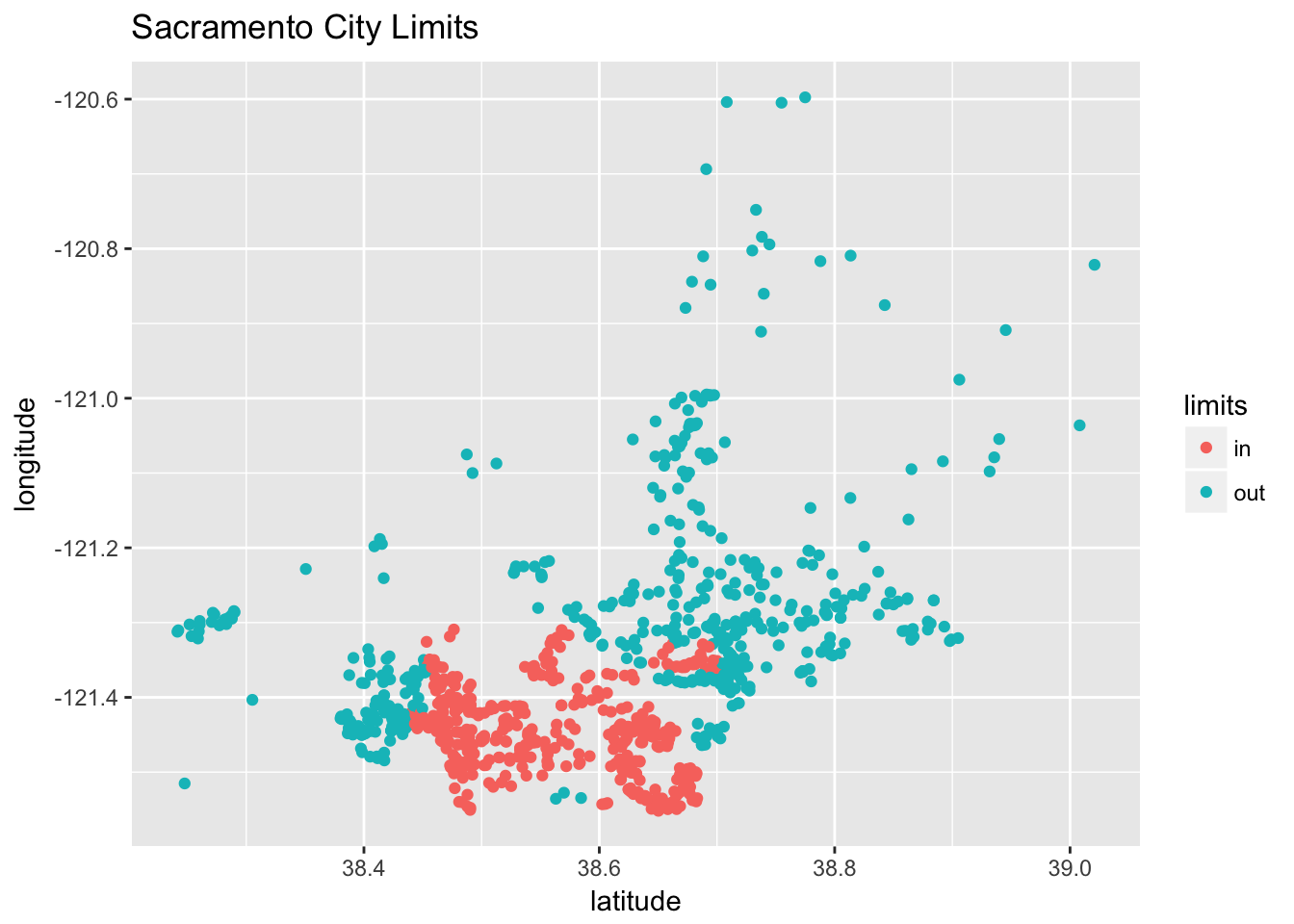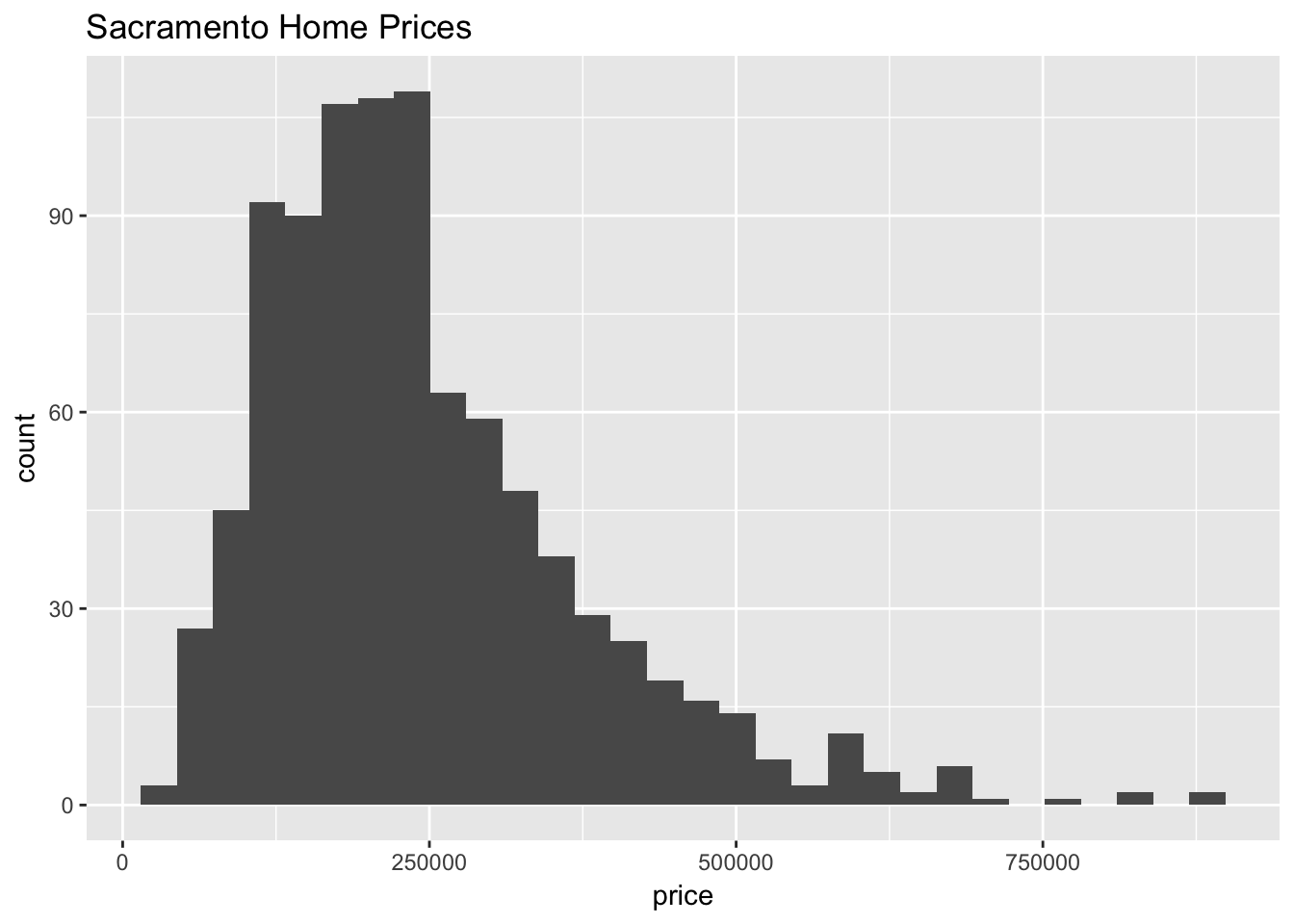Please see the homework policy document for detailed instructions and some grading notes. Failure to follow instructions will result in point reductions.

Hofstadter’s Law: “It always takes longer than you expect, even when you take into account Hofstadter’s Law.”

Douglas Hofstadter, Gödel, Escher, Bach: An Eternal Golden Braid

For this homework we will use the Sacramento data from the caret package. You should read the documentation for this data. The goal of our modeling will be to predict home prices.

You may only use the following packages:

library(caret)
library(randomForest)
library(tidyverse)
library(knitr)
library(kableExtra)

Before modeling, we will perform some data preparation.

Instead of using the city or zip variables that exist in the dataset, we will simply create a variable indicating whether or not a house is technically within the city limits Sacramento. (We do this because they would both be factor variables with a large number of factors. This is a choice that is made due to laziness, not because it is justified. Think about what issues these variables might cause.)

data(Sacramento)
sac_data = Sacramento
sac_data$limits = factor(ifelse(sac_data$city == "SACRAMENTO", "in", "out"))
sac_data = subset(sac_data, select = -c(city, zip))

A plot of longitude versus latitude gives us a sense of where the city limits are.

qplot(y = longitude, x = latitude, data = sac_data,
col = limits, main = "Sacramento City Limits ")You should consider performing some additional exploratory data analysis, but we provide a histogram of the home prices.

qplot(x = price, data = sac_data, main = "Sacramento Home Prices")After these modifications, we test-train split the data.

set.seed(42)
sac_trn_idx  = sample(nrow(sac_data), size = trunc(0.80 * nrow(sac_data)))
sac_trn_data = sac_data[sac_trn_idx, ]
sac_tst_data = sac_data[-sac_trn_idx, ]

The training data should be used for all model fitting.

## Exercise 1 (Modeling Price, Without Location)

For this exercise, we will create linear models in an attempt to be able to predict price, without the use of the limits, latitude, or longitude variables. Do not modify sac_trn_data.

With the available variables, fit the following models:

• An additive model using all availible predictors
• A model using only sqft as a predictor
• $$\texttt{price} = \beta_0 + \beta_1 \texttt{sqft} + \beta_2 \texttt{multi} + \beta_3 \texttt{res} + \epsilon$$
• $$\texttt{price} = \beta_0 + \beta_1 \texttt{sqft} + \beta_2 \texttt{multi} + \beta_3 \texttt{res} + \beta_4 (\texttt{sqft}\times\texttt{multi}) + \beta_5 (\texttt{sqft}\times\texttt{res}) + \epsilon$$

Here, res is a dummy variable indicating whether or not type is Residential. Similarly, multi is a dummy variables indicating whether or not type is Multi_Family.

Summarize these models using a well-formatted markdown table which includes columns for:

• Model (written using R’s formula syntax)
• Train RMSE
• Test RMSE

## Exercise 2 (Modeling Price, With Location)

For this exercise, we will create models in an attempt to be able to predict price, using all available predictors.

Fit a total of four models:

• add: an additive linear model using all availible predictors
• int: a linear model using all the main effects of all availible predictors as well, as all possible two-way interactions
• user: a linear model that performs better than add and int
• rf: a random forest which uses all available predictors fit using the randomForest() function from the randomForest package with all default arguments. To specify the predictors used, use the formula syntax for an additive model

Summarize these models using a well-formatted markdown table which includes columns for:

• Model Name
• Model Type (lm or rf)
• Variables Used (may use formula syntax)
• Train RMSE
• Test RMSE

## Exercise 3 (Modeling Price, Response Transformation)

Re-fit each of the models from Exercise 2, but with a log transformation applied to the response. (Do not modify the data to do so.)

Summarize the results of these four models using a well-formatted markdown table which includes columns for:

• Mode Name (append log_ to the start of the previous names)
• Model Type (lm or rf)
• Variables Used (may use formula syntax)
• Train RMSE (on the data scale, that is, units of dollars)
• Test RMSE (on the data scale, that is, units of dollars)

## Exercise 4 (Concept Checks)

[a] Which model in Exercise 1 performs best?

[b] Which model in Exercise 2 performs best?

[c] Which model in Exercise 3 performs best?

[d] Does the log transformation appear justified? Explain.

[e] Does location appear to be helpful for predicting price? Explain.

[f] Suggest a reason for performing this analysis. The reason we are creating the models is to predict price. From an analysis perspective, why might these predictions be useful?

[g] With your answer to part [f] in mind, is the best model we found at all useful? Explain.